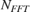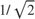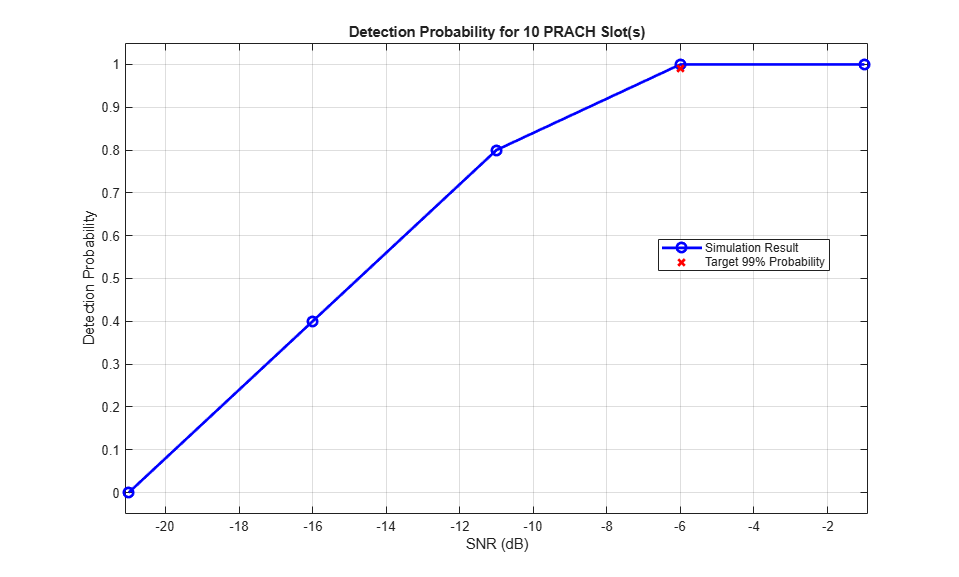# 5G NR PRACH Detection Test

This example shows how to model the physical random access channel (PRACH) missed detection conformance test, as defined in TS 38.141-1 [ 1 ]. You can learn how to measure the probability of correct detection of the PRACH preamble in the presence of a preamble signal.

### Introduction

The PRACH is an uplink transmission used by User Equipment (UE) to initiate synchronization with the gNodeB. TS 38.141-1 Section 8.4.1.5 defines the probability of PRACH detection to be greater than or equal to 99% at specific SNR values for a set of PRACH configurations and propagation conditions. There are several detection error cases:

• Detecting an incorrect preamble

• Not detecting a preamble

• Detecting the correct preamble but with the wrong timing estimation

TS 38.141-1 states that a correct detection is achieved when the estimation error of the timing offset of the strongest path is less than the time error tolerance given in Table 8.4.1.1-1. For channel propagation conditions TDLC300-100 and PRACH preamble format 0, the time error tolerance is 2.55 microseconds.

In this example, a PRACH waveform is configured and passed through an appropriate channel. At the receiver side, the example performs PRACH detection and calculates the PRACH detection probability. The example considers the parameters defined in TS 38.141-1 Table 8.4.1.5-1 and Table A.6-1. These are: normal mode (i.e., unrestricted set), 2 receive antennas, TDLC300-100 channel, normal cyclic prefix, burst format 0, SNR -6.0 dB. If you change the PRACH configuration to use one of the other PRACH preamble formats listed in Table A.6-1, you need to update the values of the time error tolerance and the SNR, according to TS 38.141-1 Table 8.4.1.1-1 and Tables 8.4.1.5-1 to 8.4.1.5-3, respectively.

### Simulation Configuration

The example considers 10 subframes at a number of SNRs. You should use a large number of numSubframes to produce meaningful results. You can set SNRdB as an array of values or a scalar. For an explanation of the SNR definition that this example uses, see SNR Definition Used in Link Simulations. Table 8.4.1.5-1 in TS 38.141-1 specifies the frequency offset foffset that is modeled between transmitter and receiver.

numSubframes = 10; % Number of 1 ms subframes to simulate at each SNR SNRdB = [-21, -16, -11, -6, -1]; % SNR range in dB foffset = 400.0; % Frequency offset in Hz timeErrorTolerance = 2.55; % Time error tolerance in microseconds 

### Carrier Configuration

Use the nrCarrierConfig configuration object carrier to specify the carrier settings. The example considers a carrier characterized by a subcarrier spacing of 15 kHz and a bandwidth of 5 MHz. That is, the carrier spans 25 resource blocks, according to Table 5.3.2-1 in TS 38.104 [ 2 ].

carrier = nrCarrierConfig; carrier.SubcarrierSpacing = 15; carrier.NSizeGrid = 25; 

### PRACH Configuration

Table A.6-1 in TS 38.141-1 specifies the PRACH configurations to use for the PRACH detection conformance test.

Set the PRACH configuration by using the nrPRACHConfig configuration object prach, according to Table A.6-1 and Section 8.4.1.4.2 in TS 38.141-1.

% Set PRACH configuration prach = nrPRACHConfig; prach.FrequencyRange = 'FR1'; % Frequency range prach.DuplexMode = 'FDD'; % Frequency Division Duplexing (FDD) prach.ConfigurationIndex = 27; % Configuration index for format 0 prach.SubcarrierSpacing = 1.25; % Subcarrier spacing prach.SequenceIndex = 22; % Logical sequence index prach.PreambleIndex = 32; % Preamble index prach.RestrictedSet = 'UnrestrictedSet'; % Normal mode prach.FrequencyStart = 0; % Frequency location % Define the value of ZeroCorrelationZone using the NCS table stored in % the nrPRACHConfig object switch prach.Format case {'0','1','2'} ncsTable = nrPRACHConfig.Tables.NCSFormat012; ncsTableCol = (string(ncsTable.Properties.VariableNames) == prach.RestrictedSet); case '3' ncsTable = nrPRACHConfig.Tables.NCSFormat3; ncsTableCol = (string(ncsTable.Properties.VariableNames) == prach.RestrictedSet); otherwise ncsTable = nrPRACHConfig.Tables.NCSFormatABC; ncsTableCol = contains(string(ncsTable.Properties.VariableNames), num2str(prach.LRA)); end NCS = 13; zeroCorrelationZone = ncsTable.ZeroCorrelationZone(ncsTable{:,ncsTableCol}==NCS); prach.ZeroCorrelationZone = zeroCorrelationZone; % Cyclic shift index % Compute the OFDM-related information for this PRACH configuration windowing = []; ofdmInfo = nrPRACHOFDMInfo(carrier,prach,'Windowing',windowing); 

### Propagation Channel Configuration

Use the nrTDLChannel object to configure the tapped delay line (TDL) propagation channel model channel as described in TS 38.141-1 Table 8.4.1.1-1.

channel = nrTDLChannel; channel.DelayProfile = "TDL-C"; % Delay profile channel.DelaySpread = 300e-9; % Delay spread in seconds channel.MaximumDopplerShift = 100.0; % Maximum Doppler shift in Hz channel.SampleRate = ofdmInfo.SampleRate; % Input signal sample rate in Hz channel.MIMOCorrelation = "Low"; % MIMO correlation channel.TransmissionDirection = "Uplink"; % Uplink transmission channel.NumTransmitAntennas = 1; % Number of transmit antennas channel.NumReceiveAntennas = 2; % Number of receive antennas channel.NormalizePathGains = true; % Normalize delay profile power channel.Seed = 42; % Channel seed. Change this for different channel realizations channel.NormalizeChannelOutputs = true; % Normalize for receive antennas 

### Loop for SNR Values

Use a loop to run the simulation for the set of SNR points given by the vector SNRdB. The SNR vector configured here is a range of SNR points including a point at -6.0 dB, the SNR at which the test requirement for PRACH detection rate (99%) is to be achieved for preamble format 0, as discussed in Table 8.4.1.5-1 in TS 38.141-1.

hNRPRACHWaveformGenerator generates an output signal normalized to the same transmit power as for an uplink data transmission within the 5G Toolbox™. Therefore, the same normalization must take place on the noise added to the PRACH. The noise added before OFDM demodulation will be amplified by the IFFT by a factor equal to the square root of the size of the IFFT (). To ensure that the power of the noise added is normalized after demodulation, and thus to achieve the desired SNR, the desired noise power is divided by. In addition, as real and imaginary parts of the noise are created separately before being combined into complex additive white Gaussian noise, the noise amplitude is scaled byso the generated noise power is 1.

At each SNR test point, calculate the probability detection on a subframe by subframe basis using these steps:

• PRACH Transmission: Use hNRPRACHWaveformGenerator to generate a PRACH waveform. Send the PRACH preambles with the timing offsets defined in TS 38.141-1 Figure 8.4.1.4.2-2. Set a timing offset base value to 50% of the number of cyclic shifts for PRACH generation. This offset is increased for each preamble, adding a step value of 0.1 microseconds, until the end of the tested range, which is 0.9 microseconds for PRACH preamble format 0. This pattern then repeats.

• Noisy Channel Modeling: Pass the waveform through a TDL channel and add additive white Gaussian noise. Add additional samples to the end of the waveform to cover the range of delays expected from the channel modeling (a combination of implementation delay and channel delay spread). This implementation delay is then removed to ensure the implementation delay is interpreted as an actual timing offset in the preamble detector.

• Application of Frequency Offset: Apply the frequency offset to the received waveform as defined by the specification.

• PRACH Detection: Perform PRACH detection using hPRACHDetect for all cell preamble indices (0-63). Use the detected PRACH index and offset returned by hPRACHDetect to determine where a detection was successful according to the constraints discussed in the Introduction section.

% Initialize variables storing probability of detection at each SNR pDetection = zeros(size(SNRdB)); % Get the maximum number of delayed samples by a channel multipath % component. This is calculated from the channel path with the largest % delay and the implementation delay of the channel filter. The example % requires this to flush the channel filter to obtain the received signal. channelInfo = info(channel); maxChDelay = ceil(max(channelInfo.PathDelays*channel.SampleRate)) + channelInfo.ChannelFilterDelay; % Total number of PRACH slots in the simulation period numPRACHSlots = floor(numSubframes / prach.SubframesPerPRACHSlot); % Store the configuration parameters needed to generate the PRACH waveform waveconfig.NumSubframes = prach.SubframesPerPRACHSlot; waveconfig.Windowing = windowing; waveconfig.Carriers = carrier; waveconfig.PRACH.Config = prach; % The temporary variables 'prach_init', 'waveconfig_init', 'ofdmInfo_init', % and 'channelInfo_init' are used to create the temporary variables % 'prach', 'waveconfig', 'ofdmInfo', and 'channelInfo' within the SNR loop % to create independent instances in case of parallel simulation prach_init = prach; waveconfig_init = waveconfig; ofdmInfo_init = ofdmInfo; channelInfo_init = channelInfo; for snrIdx = 1:numel(SNRdB) % comment out for parallel computing % parfor snrIdx = 1:numel(SNRdB) % uncomment for parallel computing % To reduce the total simulation time, you can execute this loop in % parallel by using the Parallel Computing Toolbox. Comment out the 'for' % statement and uncomment the 'parfor' statement. If the Parallel Computing % Toolbox(TM) is not installed, 'parfor' defaults to normal 'for' statement % Set the random number generator settings to default values rng('default'); % Initialize variables for this SNR point, required for initialization % of variables when using the Parallel Computing Toolbox prach = prach_init; waveconfig = waveconfig_init; ofdmInfo = ofdmInfo_init; channelInfo = channelInfo_init; % Reset the channel so that each SNR point will experience the same % channel realization reset(channel); % Normalize noise power to take account of sampling rate, which is a % function of the IFFT size used in OFDM modulation. The SNR is defined % per resource element for each receive antenna. SNR = 10^(SNRdB(snrIdx)/10); N0 = 1/sqrt(2.0*channel.NumReceiveAntennas*double(ofdmInfo.Nfft)*SNR); % Detected preamble count detectedCount = 0; % Loop for each PRACH slot numActivePRACHSlots = 0; for nSlot = 0:numPRACHSlots-1 prach.NPRACHSlot = nSlot; % Generate PRACH waveform for the current slot waveconfig.PRACH.Config.NPRACHSlot = nSlot; [waveform,~,winfo] = hNRPRACHWaveformGenerator(waveconfig); % Skip this slot if the PRACH is inactive if (isempty(winfo.WaveformResources.PRACH)) continue; end numActivePRACHSlots = numActivePRACHSlots + 1; % Set PRACH timing offset in microseconds as per TS 38.141-1 Figure % 8.4.1.4.2-2 and Figure 8.4.1.4.2-3 if prach.LRA==839 % Long preamble, values as in Figure 8.4.1.4.2-2 baseOffset = ((winfo.WaveformResources.PRACH.Resources.PRACHSymbolsInfo.NumCyclicShifts/2)/prach.LRA)/prach.SubcarrierSpacing*1e3; % (microseconds) timingOffset = baseOffset + mod(nSlot,10)/10; % (microseconds) else % Short preamble, values as in Figure 8.4.1.4.2-3 baseOffset = 0; % (microseconds) timingOffset = baseOffset + mod(nSlot,9)/10; % (microseconds) end sampleDelay = fix(timingOffset / 1e6 * ofdmInfo.SampleRate); % Generate transmit waveform txwave = [zeros(sampleDelay,1); waveform(1:(end-sampleDelay))]; % Pass data through channel model. Append zeros at the end of the % transmitted waveform to flush channel content. These zeros take % into account any delay introduced in the channel. This is a mix % of multipath delay and implementation delay. This value may % change depending on the sampling rate, delay profile and delay % spread rxwave = channel([txwave; zeros(maxChDelay, size(txwave,2))]); % Add noise noise = N0*complex(randn(size(rxwave)), randn(size(rxwave))); rxwave = rxwave + noise; % Remove the implementation delay of the channel modeling rxwave = rxwave((channelInfo.ChannelFilterDelay + 1):end, :); % Apply frequency offset t = ((0:size(rxwave, 1)-1)/channel.SampleRate).'; rxwave = rxwave .* repmat(exp(1i*2*pi*foffset*t), 1, size(rxwave, 2)); % PRACH detection for all cell preamble indices [detected, offsets] = hPRACHDetect(carrier, prach, rxwave, (0:63).'); % Test for preamble detection if (length(detected)==1) % Test for correct preamble detection if (detected==prach.PreambleIndex) % Calculate timing estimation error trueOffset = timingOffset/1e6; % (s) measuredOffset = offsets(1)/channel.SampleRate; timingerror = abs(measuredOffset-trueOffset); % Test for acceptable timing error if (timingerror<=timeErrorTolerance/1e6) detectedCount = detectedCount + 1; % Detected preamble else disp('Timing error'); end else disp('Detected incorrect preamble'); end else disp('Detected multiple or zero preambles'); end end % of nSlot loop % Compute final detection probability for this SNR pDetection(snrIdx) = detectedCount/numActivePRACHSlots; end % of SNR loop 
Detected multiple or zero preambles Detected multiple or zero preambles Detected multiple or zero preambles 

### Results

At the end of the SNR loop, the example plots the calculated detection probabilities for each SNR value against the target probability.

hPRACHDetectionResults(SNRdB, numSubframes, pDetection);### Appendix

This example uses these helper functions:

### References

1. 3GPP TS 38.141-1. "NR; Base Station (BS) conformance testing. Part 1: Conducted conformance testing." 3rd Generation Partnership Project; Technical Specification Group Radio Access Network.

2. 3GPP TS 38.104. "NR; Base Station (BS) radio transmission and reception." 3rd Generation Partnership Project; Technical Specification Group Radio Access Network.